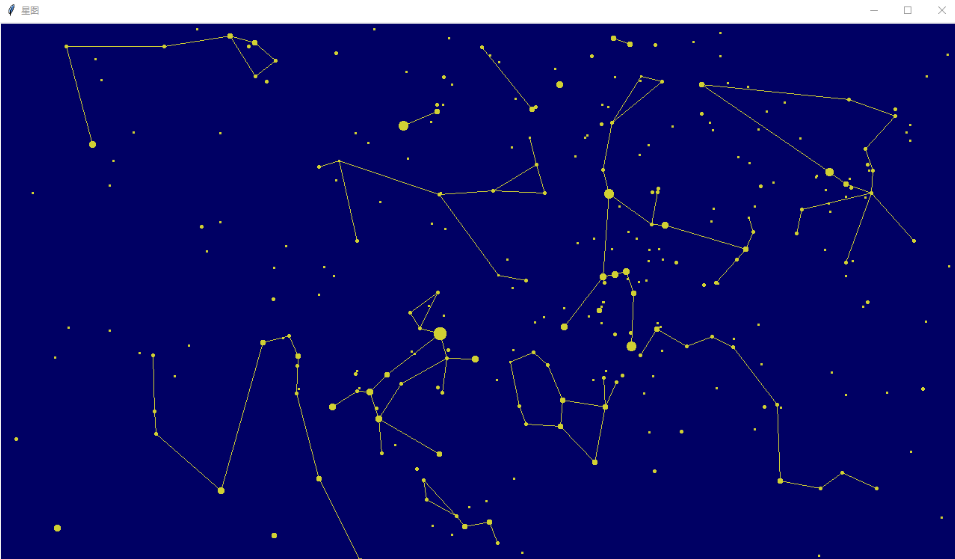# Python中的唯美星空代码详解！•  Ly   2020-06-23 17:20:08770本文讲怎样画出真实的星空。

```如果想显示真实的星空，首先你得有真实恒星的位置坐标和亮度标记。它的基本格式如下：
{'long': 0.023278328898474372, 'lat': -0.09961466705757636, 'light': 46, 'const': 66},
{'long': 0.024870941840919196, 'lat': 0.2338062439126301, 'light': 55, 'const': 62},
{'long': 0.028107061526797, 'lat': 1.1204335039257496, 'light': 56, 'const': 18},
{'long': 0.03660100303760025, 'lat': 0.5077259659824991, 'light': 21, 'const': 1},
{'long': 0.04004802831028905, 'lat': 1.0323574005393255, 'light': 23, 'const': 18},
{'long': 0.03944444109507185, 'lat': 0.3178583859888262, 'light': 55, 'const': 62},
{'long': 0.040797071265367454, 'lat': -0.488478858963941, 'light': 54, 'const': 74},
{'long': 0.0410661312228549, 'lat': -0.798444499556106, 'light': 39, 'const': 64},
{'long': 0.043800486202076855, 'lat': 0.1945266317121166, 'light': 55, 'const': 66},
{'long': 0.045036755271142, 'lat': 0.804111967609767, 'light': 50, 'const': 1},
{'long': 0.043785947609407745, 'lat': -1.4350775693910554, 'light': 53, 'const': 58},
{'long': 0.04915283505929031, 'lat': -0.2699684886295715, 'light': 49, 'const': 21},
{'long': 0.050498187206605094, 'lat': -0.4851966800391031, 'light': 54, 'const': 74},```

1、为了便于计算，首先将每颗星星的经纬度转换为xyz的三维坐标。在这种转换过程中，我们看到的是正立的天球，北极点向上，南极点在下。

2、将观测地的纬度引入每颗计算

3、将观测地经度、观测日期、观测时间三者结合起来，形成一个经度数据，引入每颗计算

4、将观测者的朝向引入每颗计算

5、将观测者的仰角引入每颗计算

6、向屏幕投影

```def calcStar(stars,Long,Lat,winLong,winLat,eyeDistant):
for star in stars:
# print(star)
# 经纬度转换为xyz的三维坐标
x0= cos(star['long'])* cos(star['lat'])
y0= sin(star['long'])* cos(star['lat'])
z0= sin(star['lat'])
# 观测地经度及日期时间的合并
x1= x0*cos(Long)- y0*sin(Long)
y1= x0*sin(Long)+ y0*cos(Long)
z1= z0
# 观测地纬度
x2= x1* sin(Lat)- z1* cos(Lat)
y2= y1;
z2= x1* cos(Lat)+ z1* sin(Lat)
# 观测者转身
x3= x2* cos(winLong)+ y2* sin(winLong)
y3= -x2* sin(winLong)+ y2* cos(winLong)
z3= z2
# 观测者俯仰
x4= x3* sin(winLat)- z3* cos(winLat)
y4= y3
z4= x3* cos(winLat)+ z3* sin(winLat)
# 屏幕投影
star['visible']= (z2> 0 and z4>0)
star['x']= x4* eyeDistant/z4
star['y']= y4* eyeDistant/z4
star['z']= z4```

```img= Image.new('RGBA', (1280,720), (0,0,120,255))  # 深蓝色天空
draw = ImageDraw.Draw(img)
color= (255,255,0,255)    # 黄色星星
for star in stars:
# 屏幕中心
x= round(-star['y']+ 640)
y= round(star['x']+ 360)
# 亮度值越小越亮，这里用大小来表示
r= round(6-star['light']/10)
if visible(star):
# print(x,y)
draw.ellipse((x-r, y-r, x+r, y+r), fill=color)
img.show()```

```def getAbsLong(aLong, ayy, amm, add, ahh, amin):
# 年的影响忽略
# 月日的影响，首先计算太阳赤经
# 春分点3月21日为经度0，一年平均365.25天，旋转360度，用插值方法简单计算
b= julianDay(ayy, 3, 21)
v= 0- (a-b)/365.25*360
# 因为使用本地时间，基本可以忽略经度+时区的影响（互相抵消）
# 时分的影响，中午12点，正对太阳赤经，一天24小时，旋转360度，用插值简单计算
c= ahh*60+amin
d= 12*60
v= v- (c-d)/(24*60)*360
return v```

```{'star1': 61, 'star2': 4, 'const': 1},
{'star1': 60, 'star2': 61, 'const': 1},
{'star1': 83, 'star2': 60, 'const': 1},
{'star1': 83, 'star2': 109, 'const': 1},
{'star1': 61, 'star2': 58, 'const': 1},
{'star1': 58, 'star2': 22, 'const': 1},
{'star1': 22, 'star2': 3097, 'const': 1},
{'star1': 3097, 'star2': 3097, 'const': 1},
{'star1': 3097, 'star2': 3088, 'const': 1},
{'star1': 3007, 'star2': 3090, 'const': 1},
{'star1': 142, 'star2': 61, 'const': 1},```